NCERT Solutions: Arithmetic Progressions (Exercise 5.3) - 2

# Arithmetic Progressions (Exercise 5.3) - 2 NCERT Solutions - Mathematics (Maths) Class 10

Q6. The first and the last terms of an AP are 17 and 350 respectively. If the common difference is 9, how many terms are there and what is their sum
Sol: We have,
First term a = 17
Last term l = 350 = Tn
Common difference d = 9
Let the number of terms be ‘n’
∵ Tn = a + (n - 1) d
∴ 350 = 17 + (n - 1) × 9
⇒ (n - 1) × 9 = 350 - 17 = 333
⇒ n - 1 = 333/9 = 37
⇒ n = 37 + 1 = 38
Since, Sn = n/2 (a + l)
∴ S38 = 38/2 (17 + 350)
= 19 (367) = 6973
Thus, n = 38 and Sn = 6973

Q7. Find the sum of first 22 terms of an AP in which d = 7 and 22nd term is 149.
Sol: Here, n = 22, T22 = 149 = l
d = 7
Let the first term of the A.P. be ‘a’.
∴ Tn = a + (n - 1) d
⇒ Tn = a + (22 - 1) × 7
⇒ a + 21 × 7 = 149
⇒ a + 147 = 149
⇒ a = 149 - 147 = 2
Now, S22 = n/2 [a + l]
⇒ S22 = 22/2 [2 + 149]
= 11  = 1661
Thus S22 = 1661

Q8. Find the sum of first 51 terms of an AP whose second and third terms are 14 and 18 respectively.
Sol: Here, n = 51, T2 = 14 and T3 = 18
Let the first term of the A.P. be ‘a’ and the common difference is d.
∴We have:
T2 = a + d ⇒ a + d = 14    ...(1)
T3 = a + 2d ⇒ a + 2d = 18   ...(2)
Subtracting (1) from 2, we get
a + 2d - a - d = 18 - 14
⇒ d = 14
From (1), we get
a + d = 14 ⇒ a + 4 = 14
⇒ a = 14 - 4 = 10
Now, Sn = n/2 [2a + (n - 1) d]
⇒ S51 = 51/2 [(2 × 10) + (51 - 1) × 4]
= 51/2 [20 + 200]
= 51/2 
= 51 × 110 = 5610
Thus, the sum of 51 terms is 5610.

Q9. If the sum of first 7 terms of an A.P. is 49 and that of 17 terms is 289, find the sum of first n terms.
Sol: Here, we have:
S7 = 49 and S17 = 289
Let the first term of the A.P. be ‘a’ and ‘d’ be the common difference, then
Sn = n/2 [2a + (n - 1) d]
⇒ S7 = 7/2 [2a + (7 - 1) d] = 49
⇒ 7 (2a + 6d) = 2 × 49 = 98
⇒ 2a + 6d = 98/7 = 14
⇒ 2 [a + 3d] = 14
⇒ a + 3d = 14/2 = 7
⇒ a + 3d = 7 ...(1)
Also, S17 = 17/2[2a + (17 - 1) d] = 289
⇒ 17/2 (2a + 16d) = 289
⇒ a + 8d = 289/17 = 17
⇒ a + 8d = 17 ...(2)
Subtracting (1) from (2), we have:
a + 8d - a - 3d = 17 - 7
⇒ 5d = 10
⇒ d =10/5 = 2
Now, from (1), we have
a + 3 (2) = 7
⇒ a = 7 - 6 = 1
Now, Sn = n/2 [2a + (n - 1) d]
= n/2 [2 × 1 + (n - 1) × 2]
= n/2 [2 + 2n - 2]
= n/2 [2n]
= n × n = n2
Thus, the required sum of n terms = n2.

Q10. Show that a1, a2, ..., an, ... form an A.P. where an is defined as below:
(i) an = 3 + 4n
(ii) an = 9 - 5n
Also find the sum of the first 15 terms in each case.
Sol: (i) Here, an = 3 + 4n
Putting n = 1, 2, 3, 4, ..... n, we get:
a1 = 3 + 4 (1) = 7
a2 = 3 + 4 (2) = 11
a3 = 3 + 4 (3) = 15
a4 = 3 + 4 (4) = 19
.....  ..... .....
an = 3 + 4n
∴ The A.P. in which a = 7 and d = 11 - 7 = 4 is:
7, 11, 15, 19, ....., (3 + 4n).
Now S15 = 15/2 [(2 × 7) + (15 - 1) × 4]
= 15/2 [14 + (14 × 4)]
= 15/2 [14 + 56]
= 15/2
= 15 × 35 = 525
(ii) Here, an = 9 - 5n
Putting n = 1, 2, 3, 4, ....., n, we get
a1 = 9 - 5 (1) = 4
a2 = 9 - 5 (2) = - 1
a3 = 9 - 5 (3) = - 6
a4 = 9 - 5 (4) = - 11
..... .....
∴ The A.P. is:
4, - 1, - 6, - 11, ..... 9 - 5 (n) [having first term as 4 and d = - 1 - 4 = - 5]
∴ S15 = 15/2 [(2 × 4) + (15 - 1) × ( - 5)]
= 15/2[8 + 14 × (- 5)]
= 15/2[8 - 70]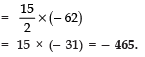Q11. If the sum of the first n terms of an AP is 4n - n2, what is the first term (that is S1)? What is the sum of first two terms? What is the second term? Similarly, find the 3rd, the 10th and the nth terms.
Sol: We have:
Sn = 4n - n2
∴ S1 = 4 (1) - (1)2
= 4 - 1 = 3 ⇒ First term = 3
S2 = 4 (2) - (2)2
= 8 - 4 = 4 ⇒ Sum of first two terms = 4
∴ Second term (S2 - S1) = 4 - 3 = 1
S3 = 4 (3) - (3)2
= 12 - 9 = 3 ⇒ Sum of first 3 terms = 3
∴ Third term (S3 - S2) = 3 - 4 = - 1
S9 = 4 (9) - (9)2
= 36 - 81 = - 45
S10 = 4 (10) - (10)2
= 40 - 100 = - 60
∴ Tenth term = S10 - S9 = [- 60] - [- 45] = - 15
Now, Sn = 4 (n) - (n)2 = 4n - n2
Also Sn - 1 = 4 (n - 1) - (n - 1)2
= 4n - 4 - [n2 - 2n + 1]
= 4n - 4 - n2 + 2n - 1
= 6n - n2 - 5
∴ nth term = Sn - Sn - 1
= [4n - n2] - [6n - n2 - 5]
= 4n - n2 - 6n + n2 + 5 = 5 - 2n
Thus,
S1 = 3 and a1 = 3
S2 = 4 and a2 = 1
S3 = 3 and a3 = - 1
a10 = - 15 and an = 5 - 2n

Q12. Find the sum of the first 40 positive integers divisible by 6.
Sol: ∵ The first 40 positive integers divisible by 6 are:
6, 12, 18, ....., (6 × 40).
And, these numbers are in A.P. such that
a = 6
d = 12 - 6 = 6 and an = 6 × 40 = 240 = l
∴ S40 = 40/2 [(2 × 6) + (40 - 1) × 6]
= 20 [12 + 39 × 6]
= 20 [12 + 234]
= 20 × 246 = 4920
OR
Sn = n/2 [a + l]
S40 = 40/2[6 + 240]
= 20 × 246 = 4920
Thus, the sum of first 40 multiples of 6 is 4920.

Q13. Find the sum of the first 15 multiples of 8.
Sol: The first 15 multiples of 8 are:
8, (8 × 2), (8 × 3), (8 × 4), ....., (8 × 15)
or 8, 16, 24, 32, ....., 120.
These numbers are in A.P., where
a = 8 and l = 120
∴ S15 = 15/2 [a + l]
= 15/2 [8 + 120]Thus, the sum of first positive 15 multiples of 8 is 960.

Q14. Find the sum of the odd numbers between 0 and 50.
Sol: Odd numbers between 0 and 50 are:
1, 3, 5, 7, ....., 49
These numbers are in A.P. such that
a = 1 and l = 49
Here, d = 3 - 1 = 2
∴ Tn = a + (n - 1) d
⇒ 49 = 1 + (n - 1) 2
⇒ 49 - 1 = (n - 1) 2
⇒ (n - 1) = 48/2 = 24
∴ n = 24 + 1 = 25
Now, S25 = 25/2[1+49]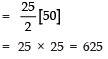Thus, the sum of odd numbers between 0 and 50 is 625.

Q15. A contract on construction job specifies a penalty for delay of completion beyond a certain date as follows: ₹ 200 for the first day, ₹ 250 for the second day, ₹ 300 for the third day, etc., the penalty for each succeeding day being ₹ 50 more than for the preceding day. How much money the contractor has to pay as penalty, if he has delayed the work by 30 days?
Sol: Here, penalty for delay on
1st day = ₹ 200
2nd day = ₹ 250
3rd day = ₹ 300
...............
...............
Now, 200, 250, 300, ..... are in A.P. such that
a = 200, d = 250 - 200 = 50
∴ S30 is given by
S30 = 30/2 [2 (200) + (30 - 1) × 50]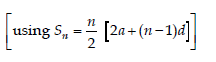= 15 [400 + 29 × 50]
= 15 [400 + 1450]
= 15 × 1850 = 27,750
Thus, penalty for the delay for 30 days is ₹ 27,750.

Q16. A sum of ₹ 700 is to be used to give seven cash prizes to students of a school for their overall academic performace. If each prize is ₹ 20 less than its preceding prize, find the value of each of the prizes.
Sol: Sum of all the prizes = ₹ 700
Let the first prize = a
∴ 2nd prize = (a - 20)
3rd prize = (a - 40)
4th prize = (a - 60)
........................................
Thus, we have, first term = a
Common difference = - 20
Number of prizes, n = 7
Sum of 7 terms Sn = 700
Since, Sn = n/2 [2a + (n - 1) d]
⇒ 700 = 7/2 [2 (a) + (7 - 1) × (- 20)]
⇒ 700 = 7/2 [2a + (6 × - 20)]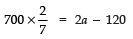⇒ 200 = 2a - 120
⇒ 2a = 200 + 120 = 320
⇒ a = 320/2 = 160
Thus, the values of the seven prizes are:
₹ 160, ₹ (160 - 20), ₹ (160 - 40), ₹ (160 - 60), ₹ (160 - 80), ₹ (160 - 100) and
₹ (160 - 120)
⇒ ₹ 160, ₹ 140, ₹ 120, ₹ 100, ₹ 80, ₹ 60 and ₹ 40.

Q17. In a school, students thought of planting trees in and around the school to reduce air pollution. It was decided that the number of trees that each section of each class will plant, will be the same as the class, in which they are studying, e.g., a section of Class I will plant 1 tree, a section of Class II will plant 2 trees and so on till Class XII. There are three sections of each class. How many trees will be planted by the students?
Sol: Number of classes = 12
∵ Each class has 3 sections.
∴ Number of plants planted by class I = 1 × 3 = 3
Number of plants planted by class II = 2 × 3 = 6
Number of plants planted by class III = 3 × 3 = 9
Number of plants planted by class IV = 4 × 3 = 12
.......................................................................................................
Number of plants planted by class XII = 12 × 3 = 36
The numbers 3, 6, 9, 12, ..........., 36 are in A.P.
Here, a = 3 and d = 6 - 3 = 3

∵ Number of classes = 12
i.e., n = 12
∴ Sum of the n terms of the above A.P., is given by
S12 = 12/2 [2 (3) + (12 - 1) 3]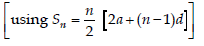= 6 [6 + 11 × 3]
= 6 [6 + 33]
= 6 × 39 = 234
Thus, the total number of trees = 234.

Q18. A spiral is made up of successive semi-circles, with centres alternately at A and B, starting with centre at A, of radii 0.5 cm, 1.0 cm, 1.5 cm, 2.0 cm, ..... as shown in figure. What is the total length of such a spiral made up of thirteen consecutive semi-circles? (Take π =22/7)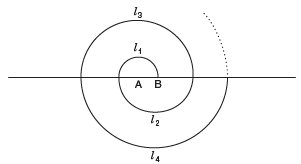[Hint: Length of successive semi-circles is l1, l2, l3, l4, ... with centres at A, B, A, B, ..., respectively.]
Sol: ∴ Length of a semi-circle = semi-circumference
= 1/2 (2πr)
= πr
∴ l1 = π r1 = 0.5 π cm = 1 × 0.5 π cm
l2 = π r2 = 1.0 π cm = 2 × 0.5 π cm
l3 = π r3 = 1.5 π cm = 3 × 0.5 π cm
l4 = π r4 = 2.0 π cm = 4 × 0.5 π cm
...... ............... ......................
l13 = π r13 cm = 6.5 π cm = 13 × 0.5 π cm
Now, length of the spiral
= l1 + l2 + l3 + l4 + ..... + l13
= 0.5 π [1 + 2 + 3 + 4 + ..... + 13] cm ...(1)
∵ 1, 2, 3, 4, ....., 13 are in A.P. such that
a = 1 and l = 13
∴ S13 = 13/2 [1+13]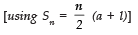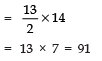∴ From (1), we have:
Total length of the spiral
=  1.5 π  cm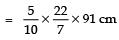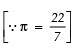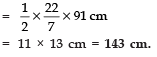Q19. 200 logs are stacked in the following manner: 20 logs in the bottom row, 19 in the next row, 18 in the row next to it and so on (see figure). In how many rows are the 200 logs placed and how many logs are in the top row?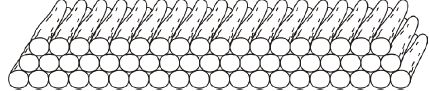Sol: We have:
The number of logs:
1st row = 20
2nd row = 19
3rd row = 18
obviously, the numbers
20, 19, 18, ....., are in A.P. such that
a = 20
d = 19 - 20 = - 1
Let the numbers of rows be n.
∴ Sn = 200
Now, using, Sn =  [2a + (n - 1) d], we get
Sn = n/2 [2 (20) + (n - 1) × (- 1)]
⇒ 200 = n/2 [40 - (n - 1)]
⇒ 2 × 200 = n × 40 - n (n - 1)
⇒ 400 = 40n - n2 + n
⇒ n2 - 41n + 400 = 0
⇒ n2 - 16n - 25n + 400 = 0
⇒ n (n - 16) - 25 (n - 16) = 0
⇒ (n - 16) (n - 25) = 0
Either
⇒ n - 16 = 0 ⇒  n = 16
or n - 25 = 0 ⇒  n = 25
Tn = 0 ⇒  a + (n - 1) d = 0 ⇒  20 + (n - 1) × (- 1) = 0
⇒ n - 1 = 20 ⇒  n = 21
i.e., 21st term becomes 0
∴ n = 25 is not required.
Thus, n = 16
∴ Number of rows = 16
Now, T16 = a + (16 - 1) d
= 20 + 15 × (- 1)
= 20 - 15 = 5
∴ Number of logs in the 16th (top) row is 5.

Q20. In a potato race, a bucket is placed at the starting point, which is 5 m from the first potato, and the other potatoes are placed 3 m apart in a straight line. There are ten potatoes in the line.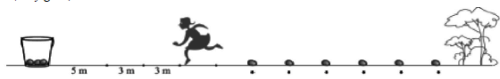A competitor starts from the bucket, picks up the nearest potato, runs back with it, drops it in the bucket, runs back to pick up the next potato, runs to the bucket to drop it in, and she continues in the same way until all the potatoes are in the bucket. What is the total distance the competitor has to run?
[Hint: To pick up the first potato and the second potato, the total distance (in metres) run by a competitor is 2 × 5 + 2 × (5 + 3)]
Sol: Here, number of potatoes = 10
The up-down distance of the bucket:
From the 1st potato = [5 m] × 2 = 10 m
From the 2nd potato = [(5 + 3) m] × 2 = 16 m
From the 3rd potato = [(5 + 3 + 3) m] × 2 = 22 m
From the 4th potato = [(5 + 3 + 3 + 3) m] × 2 = 28 m
.................................. ...........................
∵ 10, 16, 22, 28, ..... are in A.P. such that
a = 10 and d = 16 - 10 = 6
∴ Using S= n/2 [2a + (n - 1) d], we have:
S10 = 10/2[2 (10) + (10 - 1) × 6]
= 5 [20 + 9 × 6]
= 5 [20 + 54]
= 5 
= 5 × 74 = 370
Thus, the sum of above distances = 370 m.
⇒ The competitor has to run a total distance of 370 m.

The document Arithmetic Progressions (Exercise 5.3) - 2 NCERT Solutions | Mathematics (Maths) Class 10 is a part of the Class 10 Course Mathematics (Maths) Class 10.
All you need of Class 10 at this link: Class 10

## Mathematics (Maths) Class 10

115 videos|478 docs|129 tests

## FAQs on Arithmetic Progressions (Exercise 5.3) - 2 NCERT Solutions - Mathematics (Maths) Class 10

 1. What is an arithmetic progression?Ans. An arithmetic progression is a sequence of numbers in which the difference between any two consecutive terms is always the same. This difference is called the common difference.
 2. How can I find the nth term of an arithmetic progression?Ans. To find the nth term of an arithmetic progression, you can use the formula: nth term = first term + (n-1) * common difference. By substituting the values of the first term, common difference, and the value of n, you can easily calculate the nth term.
 3. Can an arithmetic progression have a negative common difference?Ans. Yes, an arithmetic progression can have a negative common difference. The common difference can be positive, negative, or zero, depending on the sequence of numbers. It simply represents the change or difference between consecutive terms.
 4. What is the sum of the first n terms of an arithmetic progression?Ans. The sum of the first n terms of an arithmetic progression can be calculated using the formula: Sum = (n/2) * (2 * first term + (n-1) * common difference). By plugging in the values of n, first term, and common difference, you can find the sum of the given terms.
 5. How can I determine if a given sequence is an arithmetic progression?Ans. To determine if a given sequence is an arithmetic progression, you need to check if the difference between any two consecutive terms is always the same. If the difference remains constant throughout the sequence, then it is an arithmetic progression. Otherwise, it may be a different type of sequence.

## Mathematics (Maths) Class 10

115 videos|478 docs|129 testsExplore Courses for Class 10 examSignup to see your scores go up within 7 days! Learn & Practice with 1000+ FREE Notes, Videos & Tests.
10M+ students study on EduRev
Track your progress, build streaks, highlight & save important lessons and more!
Related Searches

,

,

,

,

,

,

,

,

,

,

,

,

,

,

,

,

,

,

,

,

,

;